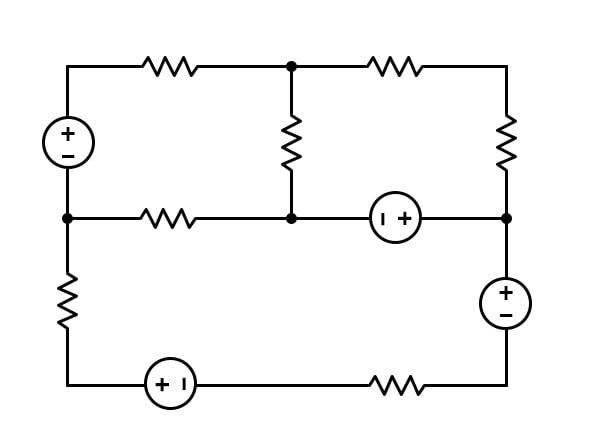# An Introduction to Electrical Energy: Current Source vs. Voltage Source

March 29, 2020 by Robert Keim
This video tutorial introduces two circuit elements that we use to represent sources of electrical energy.

Electrical engineers use electricity to accomplish useful tasks by designing circuits in which voltages and currents are controlled, modified, and utilized by a variety of different components. These voltages and currents, though, represent energy: a circuit is an energetic system in which voltage functions as potential energy and current is similar to kinetic energy. The components that we incorporate into electric circuits cannot control and utilize electrical energy unless something is also generating electrical energy, and this is why circuits have voltage sources and current sources. We call these elements sources because they supply a circuit with the electrical energy that is required for proper functionality.

## Characteristics of Voltage Sources

A voltage source generates a potential difference across its two terminals. When these two terminals are joined to a network of interconnected components that form a continuous conductive path, current will flow.

Simple electric circuits often consist of one voltage source connected to a few components. In these situations, the negative terminal of the source is interpreted as the zero-volt reference node, and consequently the node connected to the source’s positive terminal will have a voltage equal to the value of the source.However, it’s important to keep in mind that voltage sources can occupy various positions within an electrical network, and consequently, the negative terminal will not always be at 0 V. For example:Thus, the value of the source does not always indicate the voltage at the positive terminal; rather, the value of the source indicates the difference in voltage between the source’s negative terminal and the source’s positive terminal.

### Units

The value of a voltage source is the potential difference generated between the source’s two terminals, and consequently, the value is reported in volts. One volt is equal to one joule per coulomb. Thus, voltage indicates the amount of potential energy per coulomb of electric charge. To put this in practical terms, if a 5 V source and a 10 V source are connected to two identical circuits, the 10 V source propels electric charge with twice as much energy and consequently will produce twice as much current.

### Ideal Voltage Sources

When a voltage source symbol appears in a schematic, it represents an ideal voltage source. By “ideal” we mean that the voltage generated by the source never fluctuates and is not affected by the amount of current drawn by the circuit. Real-life voltage sources are never ideal.

It is often the case that this difference between theory and reality has no significant effect on circuit operation and can, therefore, be ignored. Sometimes, though, we need to introduce additional circuit elements to account for the non-ideal characteristics of real-life voltage sources.

## Characteristics of Current Sources

A current source establishes a specified amount of electric current in a portion of a circuit. The value of the current source is the magnitude of current flow created by the source, and the symbol includes an arrow to indicate the direction of the current.When you see a current source in a circuit diagram, you know that the conductive path connected to the current source will have current flow equal to the value of the source. If this path separates into multiple branches, as shown in the diagram below, you need to perform circuit analysis to determine the proportion of the source’s current that is delivered to each branch.### Units

The value of a current source is reported in amperes. This unit tells us the rate at which electric charge flows through a given point in a circuit. If we measure a current of one ampere, we know that one coulomb of charge flows past any point in the measured branch every second.

### Ideal Current Sources

An ideal current source always generates its exact rated current and is not affected by the characteristics of the circuit to which it is connected. Real-life current sources, like real-life voltage sources, are non-ideal and must be represented by an ideal source in conjunction with at least one additional component.

## Examples of Voltage and Current Sources

When we are analyzing and designing basic circuits, we see voltage sources much more frequently than current sources because electronic systems are usually powered by batteries or regulated direct-current power supplies, and both of these function as voltage sources.

We don’t typically see current sources as the primary supplier of a circuit’s electrical energy. They are more commonly a means of capturing the behavior of circuit components such as light-sensitive diodes and transistors. An exception to this is the photovoltaic cell, which is modeled as a current source (in conjunction with a few other components) and can function as the primary source of energy for a solar-powered circuit.

## Conclusion

This brief discussion of voltage and current sources has given you some basic information on these two fundamental means of introducing electrical energy into a circuit. In future video tutorials we’ll look at the difference between dependent sources and independent sources and the relationship between alternating-current (AC) voltage sources and direct-current (DC) voltage sources.